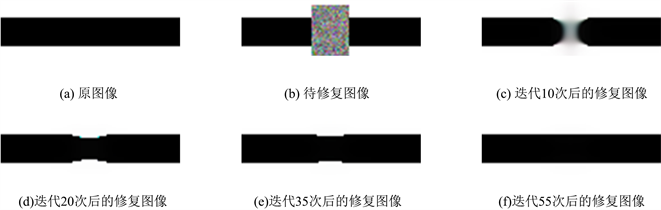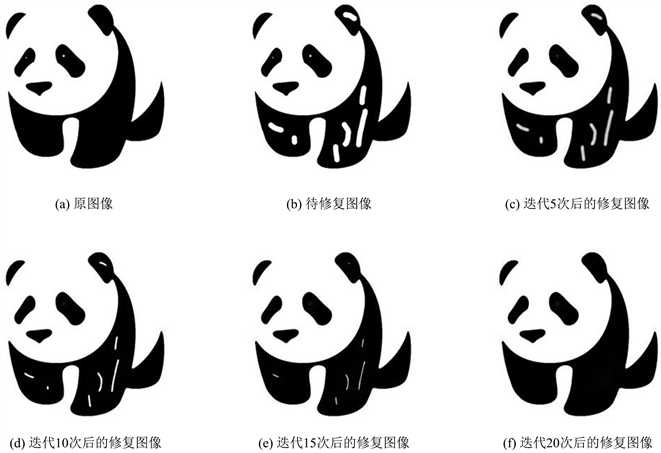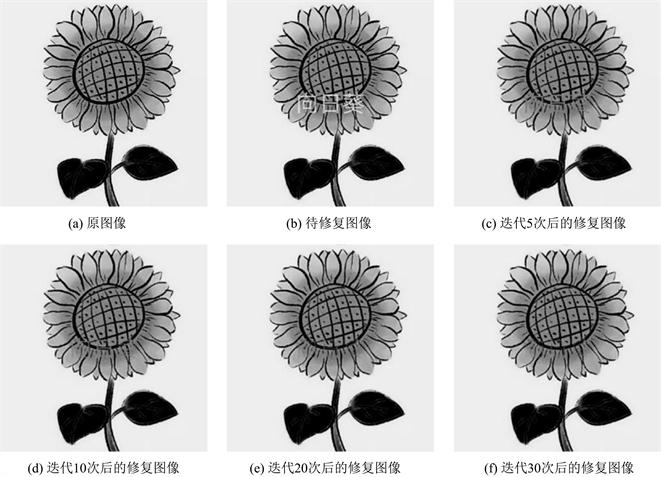﻿ 基于Cahn-Hilliard方程的二值图像修复方法

# 基于Cahn-Hilliard方程的二值图像修复方法Binary Image Inpainting Method Based on Cahn-Hilliard Equation

Abstract: As a branch of image processing, image inpainting is widely used in computer vision, astronomy, biology and other fields. In this paper, the modified Cahn-Hilliard equation is used for binary image inpainting. The second-order finite difference method is used to discretize the equation with nonlinear term in space, and the Crank-Nicolson method is used to discretize it in time. The fast discrete cosine transform combined with fixed point iteration method is used to solve the equations in the fully discrete scheme. The numerical method of image inpainting based on this model has the advantages of few parameters, small storage and high computational efficiency. Finally, numerical experiments are given to verify the effectiveness of the proposed method.

1. 引言

2. 数值方法

$\frac{\partial u}{\partial t}=\Delta \left(-\epsilon \Delta u+{\epsilon }^{-1}{W}^{\prime }\left(u\right)\right)+\lambda \left(f-u\right),\text{ }\left(x,y,t\right)\in \Omega ×\left(0,T\right].$ (1)

$\frac{\partial u}{\partial n}=\frac{\partial }{\partial n}\left(-\epsilon \Delta u+{\epsilon }^{-1}{W}^{\prime }\left(u\right)\right)=0,\text{\hspace{0.17em}}\text{\hspace{0.17em}}\text{\hspace{0.17em}}\text{\hspace{0.17em}}\left(x,y,t\right)\in \partial \Omega ×\left(0,T\right],$ (2)

$\frac{\text{d}\text{ }\mathcal{U}}{\text{d}t}=-\epsilon {K}^{2}\mathcal{U}+{\epsilon }^{-1}K{\mathcal{W}}^{\prime }\left(\mathcal{U}\right)+\lambda \left(\mathcal{F}-\mathcal{U}\right),$ (3)

$K=-\left({I}_{{N}_{y}}\otimes \Delta {x}^{-2}{B}_{{N}_{x}}+\Delta {y}^{-2}{B}_{{N}_{y}}\otimes {I}_{{N}_{x}}\right),$

${K}^{2}=\left({I}_{{N}_{y}}\otimes \Delta {x}^{-4}{B}_{{N}_{x}}^{2}+2\Delta {x}^{-2}\Delta {y}^{-2}{B}_{{N}_{y}}\otimes {B}_{{N}_{x}}+\Delta {y}^{-4}{B}_{{N}_{y}}^{2}\otimes {I}_{{N}_{x}}\right).$

${B}_{{N}_{x}}={C}_{{N}_{x}}^{\text{T}}{\Lambda }_{{N}_{x}}{C}_{{N}_{x}}={C}_{{N}_{x}}^{-\text{1}}{\Lambda }_{{N}_{x}}{C}_{{N}_{x}},$ (4)

$K=-{\left({C}_{{N}_{y}}\otimes {C}_{{N}_{x}}\right)}^{\text{T}}\Lambda \left({C}_{{N}_{y}}\otimes {C}_{{N}_{x}}\right),$ (5)(6)

3. 数值实验Figure 1. Repair of truncated bar imageFigure 2. Restoration of graffiti in imagesFigure 3. Watermark restoration in image

4. 结论

 陈永, 艾亚鹏, 郭红光. 改进曲率驱动模型的敦煌壁画修复算法[J]. 计算机辅助设计与图形学学报, 2020, 32(5): 787-796.

 谷伊, 韩军. 基于样本的图像修补方法在视频修复中的应用[J]. 应用科学学报, 2010, 28(2): 163-169.

 Gu, J., Zhang, L., Yu, G., et al. (2006) X-Ray CT Metal Artifacts Reduction through Curvature Based Sinogram Inpainting. Journal of X-Ray Science and Technology, 14, 73-82.

 Chen, X., Yang, S., Wang, X., et al. (2010) Satellite Image Blind Restoration Based on Surface Fitting and Iterative Multishrinkage Method in Redundant Wavelet Domain. Optik, 121, 1909-1911.
https://doi.org/10.1016/j.ijleo.2009.05.015

 Bertalmio, M., Sapiro, G., Caselles, V., et al. (2000) Image Inpainting. SIGGRAPH Conference, New Orleans, LA, July 2000, 417-424.
https://doi.org/10.1145/344779.344972

 杨陈东, 侯海娜. 基于改进TV修复模型的椒盐噪声去除算法[J]. 计算机与数字工程, 2016, 44(11): 2118-2123.

 Shen, J. and Chan, T.F. (2002) Mathematical Models for Local Nontexture Inpaintings. SIAM Journal on Applied Mathematics, 62, 1019-1043.
https://doi.org/10.1137/S0036139900368844

 Haehnle, J. and Prohl, A. (2011) Mumford-Shah-Euler Flow with Sphere Constraint and Applications to Color Image Inpainting. SIAM Journal on Imaging Sciences, 4, 1200-1233.
https://doi.org/10.1137/100795620

 赵颜伟, 李象霖. 基于CDD模型的快速图像修复算法[J]. 计算机仿真, 2008(10): 223-227.

 印勇, 李丁, 胡琳昀. 采用CDD模型的自适应图像修复算法[J]. 重庆大学学报, 2013, 36(4): 80-86.

 Bertozzi, A., Esedoglu, S. and Gillette, A. (2007) Analysis of a Two-Scale Cahn-Hilliard Model for Binary Image Inpainting. Multiscale Modeling & Simulation, 6, 913-936.
https://doi.org/10.1137/060660631

 Greer, J.B., Bertozzi, A.L. and Sapiro, G. (2006) Fourth Order Partial Differential Equations on General Geometries. Journal of Computational Physics, 216, 216-246.
https://doi.org/10.1016/j.jcp.2005.11.031

 Strang, G. (2007) Computational Science and Engineering. Vol. 791, Wellesley-Cambridge Press, Wellesley, 284-285.

Top